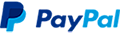Estimating Probability Distributions Course
COURSE ID: CIS532
Course Overview

In this course, you will use the Maximum Likelihood Estimate (MLE) to approximate distributions from data. Using the Bayes Optimal Classifier, you will learn how the assumptions you make will impact your estimations. You will then learn to apply the Naive Bayes Assumption to estimate probabilities for problems that contain a high number of dimensions. Ultimately, you will apply this understanding to implement the Naive Bayes Classifier in order to build a name classification system.

The course Problem-Solving with Machine Learning<.i> is required to be completed prior to starting this course.

Who should enroll in this course?
• Programmers
• Developers
• Data analysts
• Statisticians
• Data scientists
• Software engineersMachine Learning Certificate
••